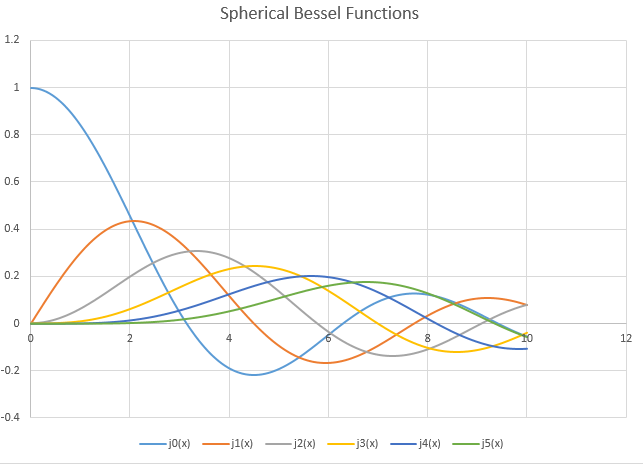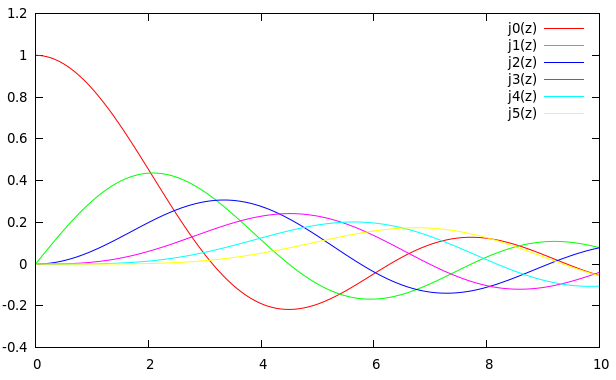# Spherical Bessel Function Integral Representation – C PROGRAM

Spherical Bessel Functions of the first kind of order n can be written in the integral form as follows:$j_n(z)=\frac{z^n}{2^{n+1}n!}\int_0^\pi \cos (z\cos \theta)\sin^{2n+1}\theta d\theta$

Using the Simpson’s Method for Numerical Integration, we can write a program that can calculate the spherical Bessel functions.

### PROGRAM:

/*******************************************
*SPHERICAL BESSEL FUNCTIONS USING INTEGRALS*
*******************************************/
#include<stdio.h>
#include<math.h>
//function to calculate the factorial
double factorial(int n){
int i;
double fact=1;
for(i=n;i>=1;i--){
fact=fact*i;
}
return fact;
}
/*Function definition to perform integration by Simpson's 1/3rd Rule */
double simpsons(double z,int n,double f(double theta,double z,int n),double a,double b,int intervals){
double h,integral,theta,sum=0;
int i;
h=fabs(b-a)/intervals;
for(i=1;i<intervals;i++){
theta=a+i*h;
if(i%2==0){
sum=sum+2*f(theta,z,n);
}
else{
sum=sum+4*f(theta,z,n);
}
}
integral=(h/3)*(f(theta,z,n)+f(theta,z,n)+sum);
return integral;
}
//function that contains the integral part of the spherical bessel function
double f(double theta,double z, int n){
return cos(z*cos(theta))*pow(sin(theta),2*n+1);
}
//function that returns the value of the spherical bessel function for a given x and n
double Jn(double z, int n){
return pow(z,n)/(pow(2,n+1)*factorial(n))*simpsons(z,n,f,0,M_PI,1000);
}

main(){
double z;
FILE *fp=NULL;
fp=fopen("sphericalBesselIntegral.txt","w");
for(z=0;z<=10;z=z+0.01){
fprintf(fp,"%lf\t%lf\t%lf\t%lf\t%lf\t%lf\t%lf\n",z,Jn(z,0),Jn(z,1),Jn(z,2),Jn(z,3),Jn(z,4),Jn(z,5));
}
}
//GNUPLOT COMMAND:
//plot 'sphericalBesselIntegral.txt' u 1:2 w l t "j0(z)", '' u 1:3 w l t "j1(z)", '' u 1:4 w l t "j2(z)", '' u 1:5 w l t "j3(z)", '' u 1:6 w l t "j4(z)", '' u 1:7 w l t "j5(z)"


### OUTPUT:

The data-points are stored in a file called sphericalBesselIntegral.txt.
They can be plotted on any software.
Copy-pasting the data to Excel and then plotting it gives the following result:One can plot the file using Gnuplot command:
plot 'sphericalBesselIntegral.txt' u 1:2 w l t "j0(z)", '' u 1:3 w l t "j1(z)", '' u 1:4 w l t "j2(z)", '' u 1:5 w l t "j3(z)", '' u 1:6 w l t "j4(z)", '' u 1:7 w l t "j5(z)" 
Output:[wpedon id="7041" align="center"]

This site uses Akismet to reduce spam. Learn how your comment data is processed.